Dr. Barry Haworth
University of Louisville
Department of Economics
Economics 202

### Closing Output Gaps with Fiscal Policy

Suppose the economy experiences an output gap whereby equilibrium GDP = 8800 and Potential GDP = 9000. This is a recessionary gap, because the equilibrium GDP is less than the Potential GDP. Our desire is to close the gap, and in order to do so, we will chose some type of fiscal policy. That is, we will either change taxes or government spending in order to close this recessionary gap of 200.

Before we proceed, let’s remind ourselves of how this economy works. Here are the equations for the various components of aggregate expenditure.

 C = 0.75(DI) + 400 (C = consumption expenditure, DI = disposable income) I = 1200 (I = investment expenditure) G = 1600 (G = government expenditure) X = 500 (X = exports) M = 600 (M = imports) T = 1200 (T = tax revenue) Yp = 9000 (Yp = potential real GDP)

From this information, we derived an equation for AE, which was AE = 0.75Y + 2200.

That is, we have aggregate expenditures (AE) that rise by 75 cents for every dollar increase in GDP (Y), but can go no lower than 2200.

If the government is using fiscal policy to close this recessionary gap, then what happens? We’ll consider three possible approaches and look at each in turn below.

(a) change (only) in government expenditure
(b) change (only) in taxes
(c) change in both government expenditure and taxes (by the same amount)

Changing Government expenditure (only)
If we use (only) government expenditure to close a recessionary gap of 200, then we do the following. The government must determine how much to change government spending (G) by in order to raise equilibrium GDP (Y*) by 200. Multiplier analysis tells us that there’s a relationship between government spending and real GDP that looks like this: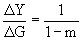Given the assumptions going into this example, we know that this relationship is constant. That is, we know that by plugging in a value for the MPC and either the change in Y or change in G, we can get a solution for the remaining variable.

What do we know here? We know that we want to increase Y by 200 and that the MPC = 0.75. Plugging those values into the government expenditure multiplier above we get: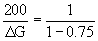Solving for DG, we get DG = 50. That is, a \$50 increase in government expenditure will close a recessionary gap of \$200.

Changing Taxes (only)
Using (only) taxes to close a recessionary gap of 200, then we do the following. As before, the government must determine how much to change taxes (T) by in order to raise equilibrium GDP (Y*) by 200. Multiplier analysis tells us that there’s a relationship between taxes and real GDP, as well, which looks like this: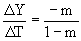Given the assumptions going into this example, we know that this relationship is constant. That is, we know that by plugging in a value for the MPC and either the change in Y or change in T, we can get a solution for the remaining variable.

What do we know here? We know that we want to increase Y by 200 and that the MPC = 0.75. Plugging those values into the government expenditure multiplier above we get: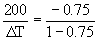Solving for DT, we get DT = -200/3. That is, a \$200/3 decrease in taxes (about \$66.67) will close a recessionary gap of \$200. If the economy includes 67 people, then this result implies that each of the 67 individuals should pay about \$1 less per period.

Changing government expenditure and taxes by the same amount
If the government decides to change taxes and government expenditure by the same amount to close this recessionary gap of 200, then our approach is a little different from what was described with taxes (only) and government expenditure (only). When G and T change by the same amount, we talk about actions designed to keep the budget balanced. Therefore, it’s relevant to ask about the net effect of changing G and T by the same amount, which leads to a discussion of the balanced budget multiplier.

What is the combined effect of changing G and T by the same amount? G is expansionary, and T is contractionary. Because G affects GDP directly and T indirectly, we expect a net GDP increase from increasing G and T by the same amount (and a net GDP decrease from decreasing G and T by the same amount). If we simply add the two multipliers together, and assume that DG = DT, we get the balanced budget multiplier.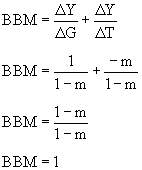What this result tells us is that any equal increase (or decrease) in government spending and taxes will cause the same increase (or decrease) in equilibrium GDP. In this situation, all we need to do is to change G and T by the amount of the recessionary gap.

That is, if government spending and taxes both increase by \$200, then equilibrium GDP will increase by \$200 as well.